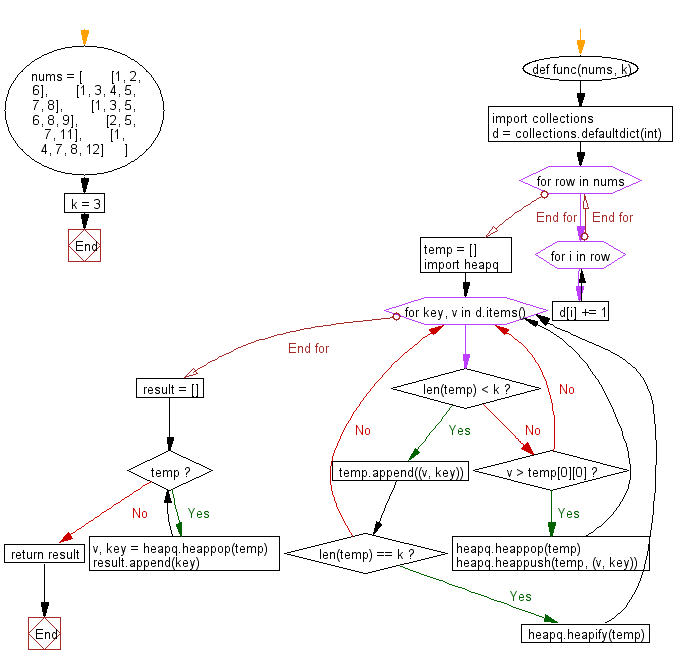﻿ Python heap queue algorithm: Find the top k integers that occur the most frequently from a given lists of sorted and distinct integers using Heap queue algorithm - w3resource

# Python: Find the top k integers that occur the most frequently from a given lists of sorted and distinct integers using Heap queue algorithm

## Python heap queue algorithm: Exercise-9 with Solution

Write a Python program to find the top k integers that occur the most frequently from a given list of sorted and distinct integers using the heap queue algorithm.

Sample Solution:

Python Code:

``````def func(nums, k):
import collections
d = collections.defaultdict(int)
for row in nums:
for i in row:
d[i] += 1
temp = []
import heapq
for key, v in d.items():
if len(temp) < k:
temp.append((v, key))
if len(temp) == k:
heapq.heapify(temp)
else:
if v > temp:
heapq.heappop(temp)
heapq.heappush(temp, (v, key))
result = []
while temp:
v, key = heapq.heappop(temp)
result.append(key)
return result
nums = [
[1, 2, 6],
[1, 3, 4, 5, 7, 8],
[1, 3, 5, 6, 8, 9],
[2, 5, 7, 11],
[1, 4, 7, 8, 12]
]
k = 3
print("Original lists:")
print(nums)
print("\nTop 3 integers that occur the most frequently in the said lists:")
print(func(nums, k))
```
```

Sample Output:

```Original lists:
[[1, 2, 6], [1, 3, 4, 5, 7, 8], [1, 3, 5, 6, 8, 9], [2, 5, 7, 11], [1, 4, 7, 8, 12]]

Top 3 integers that occur the most frequently in the said lists:
[5, 7, 1]
```

Flowchart:Python Code Editor:

Have another way to solve this solution? Contribute your code (and comments) through Disqus.

What is the difficulty level of this exercise?

Test your Programming skills with w3resource's quiz.

﻿